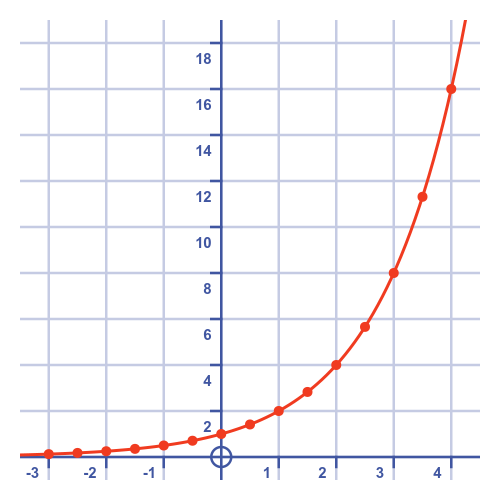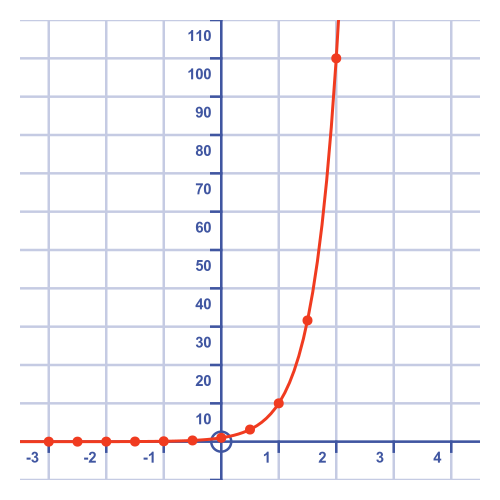# Graphs of exponential functions

By Martin McBride, 2023-02-10
Tags: exponential exponent power root graphs
Categories: exponentialsWe can calculate the value of ax for any rational value of x, where a is a positive value, as described here. We can also find the value of ax for irrational values of x as shown here.

This allows us to plot a graph of the exponential function for any positive value of a.

## Exponential function with base 2

This graph shows the function $y = 2^x$:It shows values of x from -3 to 3, in steps of 1/2. These steps can be calculated as described above for fractional exponents.

As with all exponential graphs, the graph gets steeper as x increases

## Exponential function with base 10

This graph shows the function $y = 10^x$:Notice that this graph is a lot steeper than the graph of $y = 2^x$. The two graphs have different x-scales.